# detectRUNS: an R package to detect runs of homozygosity and heterozygosity in diploid genomes

IBBA-CNR, PTP, Università degli Studi di Sassari, University of Guelph
,

### 2019-10-24

## Using detectRUNS 0.9.6

# Overview

detectRUNS is a R package for the detection of runs of homozygosity (ROH/ROHom) and of heterozygosity (ROHet, a.k.a. “heterozygosity-rich regions”) in diploid genomes. ROH/ROHom were first studied in humans (e.g. McQuillan et al. 2008) and rapidly found applications not only in human genetics abut also in animal genetics (e.g. Ferencakovic et al., 2011, in Bos taurus). More recently, the idea of looking also at “runs of heterozygosity” (ROHet or, more appropriately, “heterozygosity-rich regions”) has been proposed (Wiliams et al. 2016).

detectRUNS uses two methods to detect genomic runs:

1. sliding-window based method:
2. consecutive runs:

The sliding-window based method is similar to what is implemented in the computer package Plink (Purcell et al., 2007) [see Bjelland et al., 2013 for a description]. In brief, a sliding window is used to scan the genome, and the characteristics of consecutive windows are used to determine whether a SNP is or not in a run (either ROH/ROHom or ROHet). Parameters for both the sliding window and the run need to be specified.

The “consecutive runs” method is window-free and directly scans the genome SNP by SNP. It was first proposed by Marras et al. (2015). Here, only parameters for the runs need to be specified.

Besides detecting genomic runs (again, either homozygosity or heterozygosity, either sliding-window based or consecutive), and saving results to a data frame of individual runs, detectRUNS can:

• plot runs along the genome:
• plot runs per individual
• plot stacked (piled) runs
• plot the % of times each SNP is in a run in the population (per chromosome)
• Manhattan-like plot of the % of times each SNP is in a run
• plot mean or total run length vs number of runs per individual
• generate summary descriptive statistics on detected runs
• calculate inbreeding based on ROH ($$F_{ROH}$$), genome-wide and chromosome-wide
• plot $$F_{ROH}$$ per chromosome

The input files for detectRUNS are Plink ped/map files. If one wishes to use this R package only for plots and summary statistics, output files from Plink (.hom files) can be easily read into detectRUNS through a specific function.

detectRUNS can be used with genotype data from any diploid organisms: humans, animals or plants.

# Sample data

To illustrate the functionalities of detectRUNS, we use data on sheep (Ovis aries) SNP genotypes from the work by Kijas et al. (2016), available on-line through “Dryad” (https://goo.gl/sfAy8k). A subset with two breeds (“Jacobs” and “Navajo-Churro”, 100 animals) and two chromosomes ($$4\,841$$ SNPs from OAA 2 and 24) was used.

genotypeFilePath <- system.file(
"extdata", "Kijas2016_Sheep_subset.ped", package="detectRUNS")
mapFilePath <- system.file(
"extdata", "Kijas2016_Sheep_subset.map", package="detectRUNS")

# Detect runs

For the detection of genomic runs, detectRUNS uses two main functions:

1. slidingRUNS.run: for sliding-window-based detection
2. consecutiveRUNS.run: for consecutive-SNP-based detection

Input files are to be passed as paths to files (e.g. /home/Documents/experiment/file.ped/map).

## sliding-window-based run detection

The function slidingRUNS.run() accepts in input several parameters: besides the paths (or names) of ped/map files, there are parameters related to the sliding window and parameters related to the genomic runs.

Sliding-window parameters are: windowSize, threshold (to call a SNP “in run”), minSNP (minimum number of homozygous/heterozygous SNP in the window), maxOppWindow (maximum number of SNP with opposite genotype: heterozygous/homozygous) and maxMissWindow (maximum number of missing genotypes).

Run-related parameters are: maxGap (maximum gap between consecutive SNPs, in basepairs -bps), minLenghtBps (minimum length of the run, in bps), minDensity (number of SNPs every $$x$$ kilo-basepairs -kbps), maxOppRun (maximum number of opposite genotypes in the run), maxMissRun (maximum number of missing genotypes in the run).

ROHet controls whether runs of homozygosity (ROH/ROHom) or of heterozygosity (heterozygosity-rich regions, ROHet) will be detected. It defaults to FALSE (ROH/ROHom).

slidingRuns <- slidingRUNS.run(
genotypeFile = genotypeFilePath,
mapFile = mapFilePath,
windowSize = 15,
threshold = 0.05,
minSNP = 20,
ROHet = FALSE,
maxOppWindow = 1,
maxMissWindow = 1,
maxGap = 10^6,
minLengthBps = 250000,
minDensity = 1/10^3, # SNP/kbps
maxOppRun = NULL,
maxMissRun = NULL
) 

## consecutive SNP-based run detection

The function consecutiveRUNS.run() has a similar structure, obviously without the sliding-window parameters.

consecutiveRuns <- consecutiveRUNS.run(
genotypeFile =genotypeFilePath,
mapFile = mapFilePath,
minSNP = 20,
ROHet = FALSE,
maxGap = 10^6,
minLengthBps = 250000,
maxOppRun = 1,
maxMissRun = 1
)

slidingRUNS.run() detected 1348 ROH; consecutiveRUNS.run() detected 1144 ROH.

## “Runs of heterozygosity” (a.k.a. heterozygosity-rich regions)

By setting ROHet=TRUE, runs of heterozygosity (a.k.a. heterozygosity-rich genomic regions) are detected instead. Again, the usert can choose whether to use the sliding-window or the consecutive method.

slidingRuns_het <- slidingRUNS.run(
genotypeFile = genotypeFilePath,
mapFile = mapFilePath,
windowSize = 10,
threshold = 0.05,
minSNP = 10,
ROHet = TRUE,
maxOppWindow = 2,
maxMissWindow = 1,
maxGap = 10^6,
minLengthBps = 10000,
minDensity = 1/10^6, # SNP/kbps
maxOppRun = NULL,
maxMissRun = NULL
) 
consecutiveRuns_het <- consecutiveRUNS.run(
genotypeFile =genotypeFilePath,
mapFile = mapFilePath,
minSNP = 10,
ROHet = TRUE,
maxGap = 10^6,
minLengthBps = 10000,
maxOppRun = 2,
maxMissRun = 1
)

slidingRUNS.run() detected 2297 ROHet; consecutiveRUNS.run() detected 1870 ROHet.

Runs of homozygosity (ROH) detected using the sliding-windows method (output from slidingRUNS.run()) will be used to illustrate summary statistics, plots and inbreeding calculations.

# Summary statistics on detected runs

The function summaryRuns() takes in input the dataframe with results from runs detection and calculates a number of basic descriptive statistics on runs. Additional necessary parameters are the paths to the Plink ped and map files. Class and snpInRuns are optional arguments.

summaryList <- summaryRuns(
runs = slidingRuns, mapFile = mapFilePath, genotypeFile = genotypeFilePath,
Class = 6, snpInRuns = TRUE)

The returned list includes the following dataframes:

summary_ROH_count_chr, summary_ROH_percentage_chr, summary_ROH_count, summary_ROH_percentage, summary_ROH_mean_chr, summary_ROH_mean_class, result_Froh_genome_wide, result_Froh_chromosome_wide, result_Froh_class, SNPinRun

We can, for instance, have a look at the number of runs per class-size (Mbps) in the two breeds: we see that in Jacobs sheep there are 894 ROH with size up to 6 Mbps.

summaryList$summary_ROH_count ## Jacobs Navajo-Churro ## 0-6 894 260 ## 6-12 101 20 ## 12-24 34 12 ## 24-48 14 7 ## >48 3 3 Or, the average number of ROH per chromosome and per breed can be obtained. summaryList$summary_ROH_mean_chr
##   chrom   Jacobs Navajo-Churro
## 1     2 4.069597      4.492243
## 2    24 3.697987      2.745963

The dataframe “SNPinRun” contains, for each SNP, the proportion of times it falls inside a run in any given population/group:

head(summaryList$SNPinRun) ## SNP_NAME CHR POSITION COUNT BREED PERCENTAGE ## 1 s47174.1 2 158066 11 Jacobs 17.1875 ## 2 s30876.1 2 167018 11 Jacobs 17.1875 ## 3 s16671.1 2 354035 12 Jacobs 18.7500 ## 4 s31991.1 2 544617 12 Jacobs 18.7500 ## 5 s33481.1 2 679324 12 Jacobs 18.7500 ## 6 s49858.1 2 901838 12 Jacobs 18.7500 The summary information included in the list returned from summaryRuns() is conveniently organized in data.frames, so that it can easily be visualized, manipulated and written out to text files (e.g. .csv files). # Plots detectRUNS produces a number of plots from the dataframe with runs (results from sliding-window or consecutive scans of the genome for ROH/ROHet). The basic plot, produced by the function plot_Runs(), plots directly all runs detected in each individual against their position along the chromosome. Separate plots per chromosome are produced, and different groups/populations are coloured differently to visualize contrasting patterns. plot_Runs(runs = slidingRuns)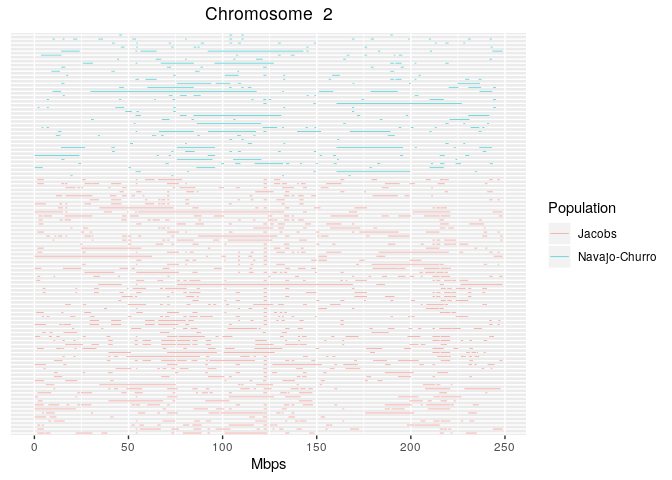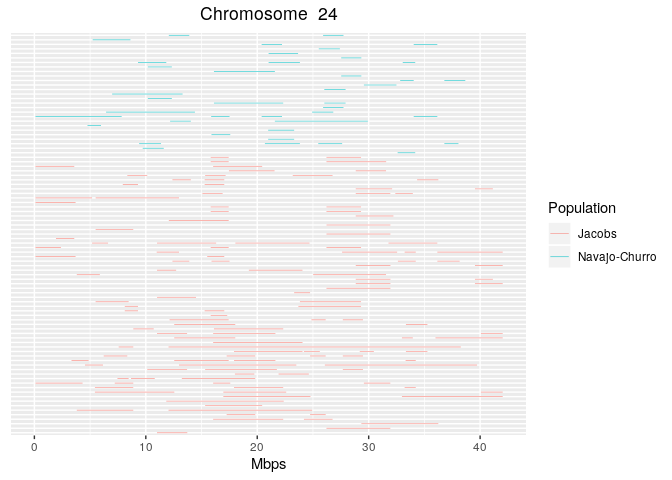Alternatively, runs can still be plotted against their position along the chromosome, but stacked on top of each other: this way, regions of the genome with an excesse of runs can easily be identified. In this case, separate plots per chromosome and per group/population are produced. plot_StackedRuns(runs = slidingRuns)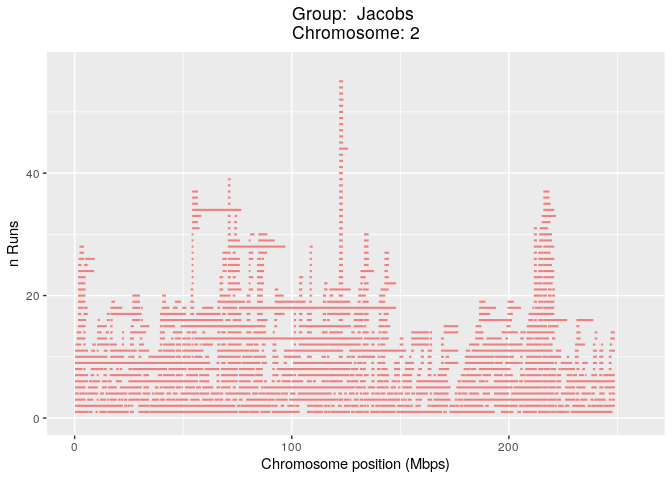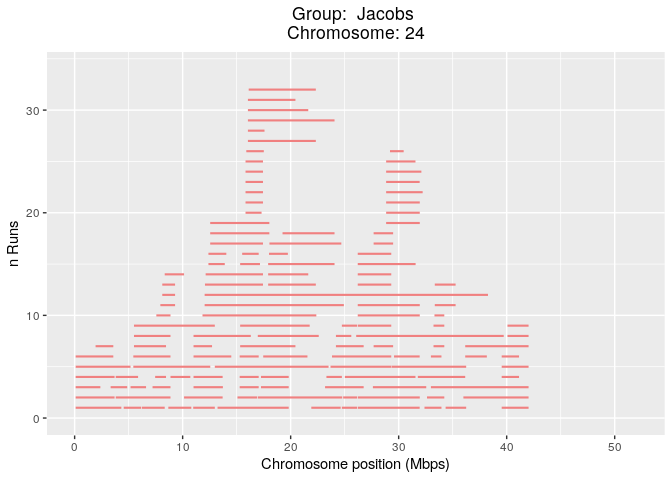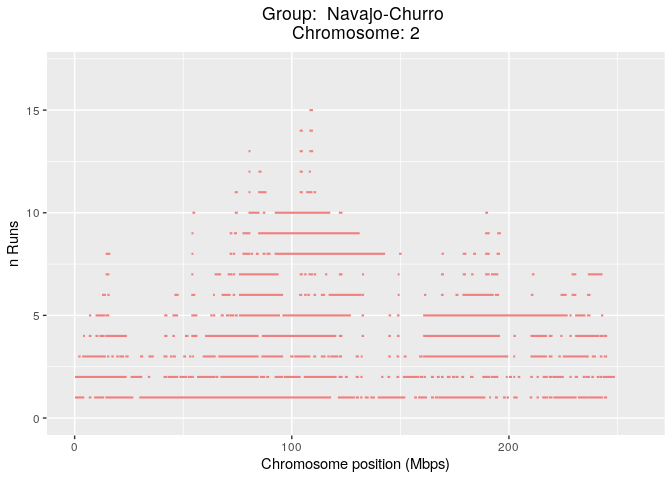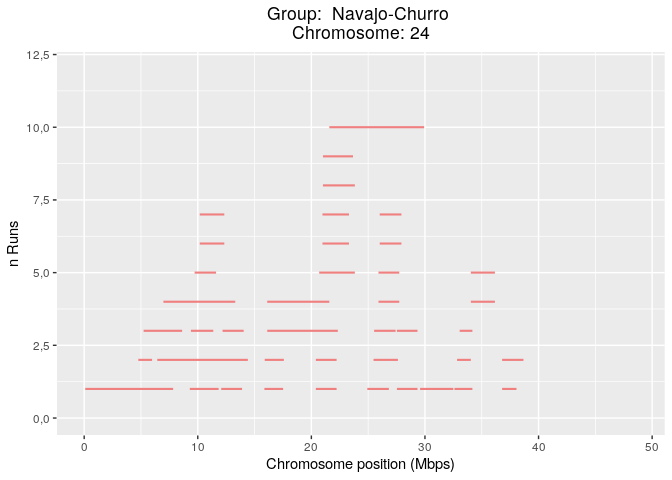Finally, the proportion of times each SNP falls inside a run in any given population/group can be plotted against their position along the chromosome, separately per group. The function plot_SnpsInRuns() requires as arguments, besides the dataframe with detected runs, also the paths to the original ped (for information on groups) and map (for SNP positions) files. plot_SnpsInRuns( runs = slidingRuns[slidingRuns$chrom==2,], genotypeFile = genotypeFilePath,
mapFile = mapFilePath)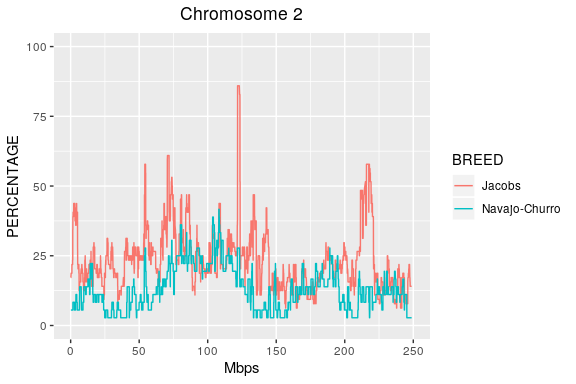plot_SnpsInRuns(
runs = slidingRuns[slidingRuns$chrom==24,], genotypeFile = genotypeFilePath, mapFile = mapFilePath)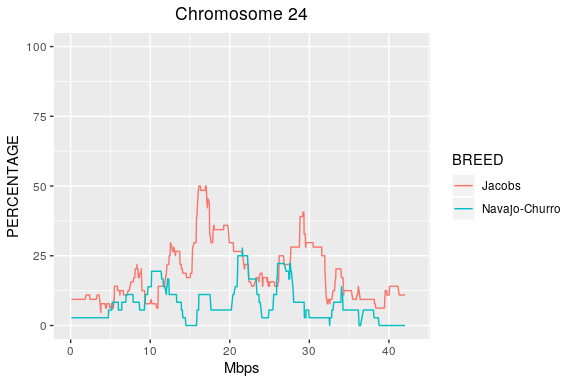We can see from the plots above, that in the Jacob sheep breed a region with a “peakk”" of ROH can be spotted approximately halfway on chromosome 2 (OAR2) in the Jacob breed. This corresponds to the strong GWAS signals found by Kijas et al. (2016) on OAR2 associated with the four-horns phenotype. To identify the position of a runs (ROH in this case) peak, e.g. from plot_SnpsInRuns(), one can conveniently use the function detectRUNS::tableRuns(): this requests as input, besides the runs dataframe, also the paths to the original ped/map files, and the threshold above which we want information on such “peaks” (e.g. only peaks where SNP are inside runs in more than 70% of the individuals in that population/group). topRuns <- tableRuns( runs = slidingRuns, genotypeFile = genotypeFilePath, mapFile = mapFilePath, threshold = 0.7) ## Group Start_SNP End_SNP chrom nSNP from to ## 1 Jacobs OAR2_130215973.1 OAR2_131721845.1 2 21 121796989 123528595 The information on the proportion of times each SNP falls inside a run, can also be plotted against SNP positions in all chromosomes together, similarly to the familiar GWAS Manhattan plots: plot_manhattanRuns( runs = slidingRuns[slidingRuns$group=="Jacobs",],
genotypeFile = genotypeFilePath, mapFile = mapFilePath)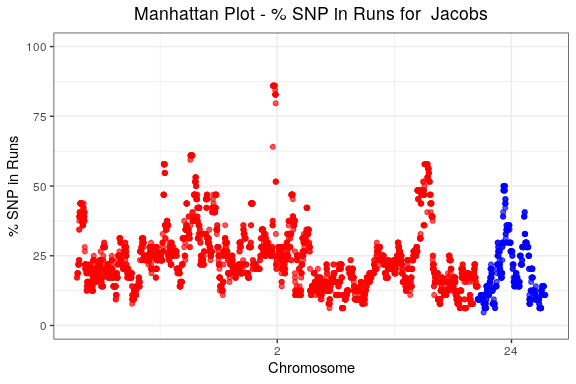# $$F_{ROH}$$: ROH-based inbreeding

From runs of homozygosity (ROH), individual inbreeding/consanguinity coefficients can be calculated as:

$F_{ROH} = \frac{\sum L_{ROH}}{L_{genome}}$

where $$\sum L_{ROH}$$ is the sum of the length of all ROH detected in an individual, and $$L_{genome}$$ is the total length of the genome that was used.

detectRUNS provide functions to calculate individual inbreeding/consanguinity

##     id         group      sum Froh_genome
## 1   H1 Navajo-Churro 16593339  0,05705571
## 2  H10 Navajo-Churro 45444521  0,15625966
## 3  H11 Navajo-Churro 18389476  0,06323168
## 4 H114        Jacobs 71163071  0,24469215
## 5 H115        Jacobs 64303071  0,22110424
## 6 H116        Jacobs 62350601  0,21439073

The parameter “genome_wide” (which defaults to TRUE) can be used to obtain inbreeding/consanguinity estimates on a per-chromosome basis (by setting “genome_wide=FALSE”)

Inbreeding levels can be plotted by group, for example: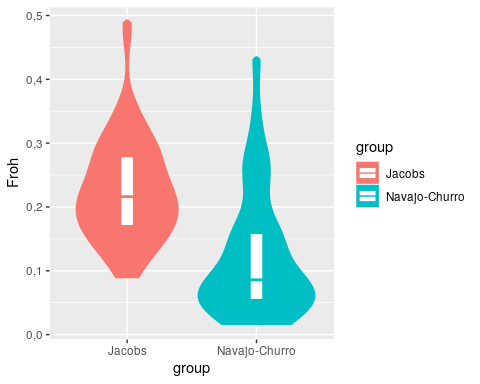# Importing data from external files

Results on runs (typically ROH) from external software can be imported into detectRUNS to produce plots, tables and summary statistics. Current options include:

• ROH from Plink (.hom file from --homozyg)
• ROH from BCFtools (output from the roh option)
• runs dataframes from detctRUNS written out to files

Through the parameter program the user can select from which source the output file is coming. As an illustration, we read in results from detectRUNS saved out to a .csv file:

savedRunFile <- system.file(
"extdata", "Kijas2016_Sheep_subset.sliding.csv", package="detectRUNS")
runs <- readExternalRuns(inputFile = savedRunFile, program = "detectRUNS")
head(runs)
##    group   id chrom nSNP     from       to lengthBps
## 1 Jacobs H114     2   76  1606489  5215635   3609146
## 2 Jacobs H114     2   44  5798140  8518359   2720219
## 3 Jacobs H114     2  148 21979508 30849683   8870175
## 4 Jacobs H114     2   45 35582012 38744795   3162783
## 5 Jacobs H114     2   15 39198369 40023789    825420
## 6 Jacobs H114     2   16 52327990 53043790    715800